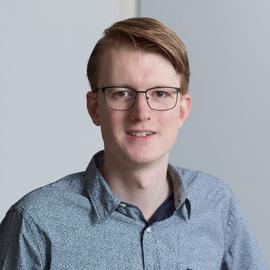# Solving LPN using Large Covering Codes

### Abstract

Learning Parity with Noise (LPN) is a computational problem that we can use for cryptographic algorithms. It is suspected that LPN can not be solved (much) more efficiently on a quantum computer than on a classic machine. The most time-efficient solving algorithms for LPN use as much memory as they need time. The amount of memory needed may be a more limiting factor for practical attacks than the time that would be spent. We propose to improve the theoretical performance of algorithms that use a reduction based on covering codes. By applying the StGen codes proposed by Samardjiska and Gligoroski, we are able to create codes that have a better bias. However, we also show that it is important to consider the time needed to decode such codes. We also study the Gauss solving algorithm, proposed by Esser, Kübler and May. It does not have the best performance, but it is able to solve LPN problems using small amounts of memory. We combine it with the code reduction to obtain a solving algorithm we will refer to as Coded Gauss. This combination should not consume too much memory and provide better performance than Gauss. Unfortunately, by applying the theoretical bounds of covering codes, we show that this combination will not work. Coded Gauss would be a less efficient algorithm than applying Gauss to the full problem. We also show that there do exist some edge-case scenarios where Coded Gauss may still be faster than Gauss, but would consume about as much memory as faster solving algorithms. Finally, we present a software implementation of algorithms that reduce and solve LPN problems. This software is easily adaptable to any attack on an LPN problem to study their behaviour. We hope that the software is helpful for people trying to understand the LPN problem and the many proposed algorithms.

Type
Publication
Master’s thesis

Master’s thesis written under the supervision of Simona Samardjiska.

## Software##### Thom Wiggers
###### PhD candidate at Radboud University

My research interests include (post-quantum) cryptography and protocols